×
Get Full Access to Penn State - PHYS 211 - Study Guide - Final
Get Full Access to Penn State - PHYS 211 - Study Guide - Final

×

PENN STATE / Physics / PHYS 211 / What are rotational dynamics?

# What are rotational dynamics? Description

PHYS 211 Final Study Guide

## What are rotational dynamics?Study guide is most useful with my notes that give highly important diagrams I will also skip useless and unimportant subchapters so you save a lot of time studying from my study guide. This study guide highlights only the important parts of my notes, so you save time​. ____________________________________________________________________________ Equations to know how to use:

1. Fnet = ma

2. Tnet = Iα (similar to Fnet)

3. I = mr2

4. L = Iw

5. Krot = ½mr2w2 = ½Iw2

## What is an example of static equilibrium?6. wf = wit + αt

7. θ = wit + ½αt2

8. wf2 = wi2 + 2αtθ

9. T = rFsin(θ)

10. Tgrav = -mgxcom

11. Fg = (GMm)/r2

12. Fs = usn

13. Fk = ukn

14. Fr = urn

## How is angular momentum defined?15. ac = v2/r = w2r Don't forget about the age old question of How do you calculate the weighted average cost of capital?

16. Fs = Static Friction

17. Fk = Kinetic Friction

18. Fr = Rolling Friction

19. Fg = Force of Gravity

G = Gravitational constant

T = Torque

Tgrav = Gravitational Torque (Torque caused by gravity)

I = Moment of Inertia

L = Angular Momentum

α = Angular Acceleration

w = Angular Velocity

θ = Angle

____________________________________________________________________________

If you really want a good grade, go through all of my other study guides listed on my StudySoup account. You will get an A then.

Chapter 12 - Rotation of a Rigid Body:

12.1 Rotational Motion: Don't forget about the age old question of How does territoriality become adaptive?
Don't forget about the age old question of Who own factors of production?

Rigid Body- An object whose size and shape does not change as it moves Rotational Kinematics:

1) wf = wit + αt

2) θ = wit + ½αt2

3) wf2 = wi2 + 2αtθ

12.2 Rotation About the Center of Mass:

Xcom = center of mass on x-axis = (m1x1 + m2x2 + m3x3 + …) / (m1 + m2 + m3 + …) Ycom = center of mass on y-axis = (m1y1 + m1y2 + m1y3 + …) / (m1 + m2 + m3 + …)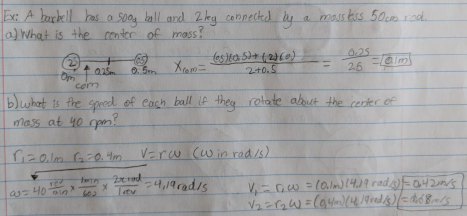12.3 Rotational Energy: We also discuss several other topics like Where is antitragus located?

Kinetic Energy due to rotation > Rotational Kinetic Energy (pretty straightforward) Krot = ½m1r12w + ½m2r22w + ½m3r32w + …

Moment of Inertia = I = m1r12 + m2r22 + …

-Object’s I depends on the axis of rotation, because that changes the r values Krot = ½Iw2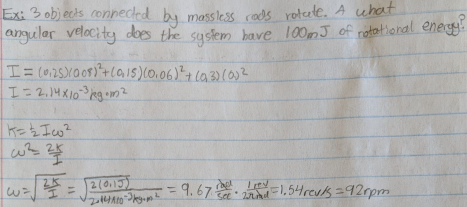Objects with larger moment of inertia are harder to rotate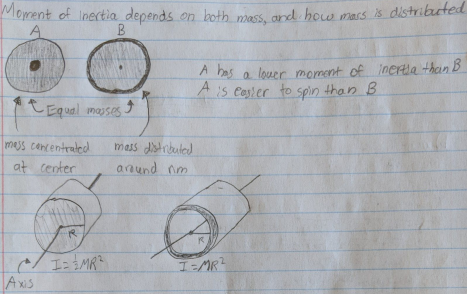Emech = Krot + Ug = ½Iw2 + MgycomIf you want to learn more check out What does the circular flow model demonstrate in Economics?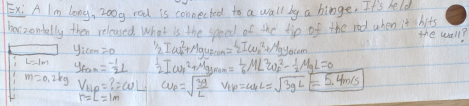12.4 Calculating Moment of Inertia: We also discuss several other topics like What is the power of media?

Parallel Axis Theorem:

The momentum of inertia depends upon the axis of rotation

To find the moment of inertia of an off center axis: I = Icom + Md2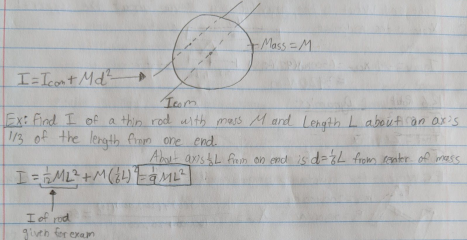12.5 Torque:

The ability of a force to a cause a rotation depends on three factors:

1) Magnitude of the Force, F

2) Distance, r, from the point of application to the pivot

3) Angle at which the force is applied

a) Angle is measured counterclockwise from the dashed line of the rotation of axis All these factors add up to create Torque​, T

T = rFsin(θ)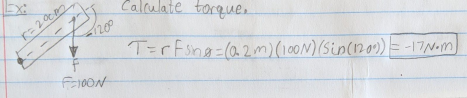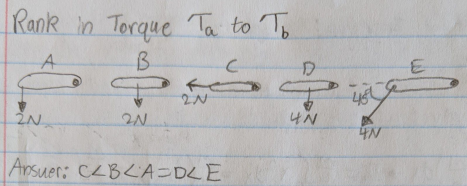Gravitational Torque = Tgrav = -Mgxcom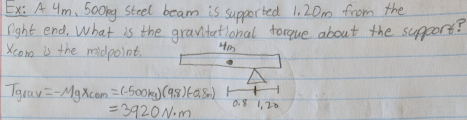12.6 Rotational Dynamics:

T = mr2α

Tnet =Iα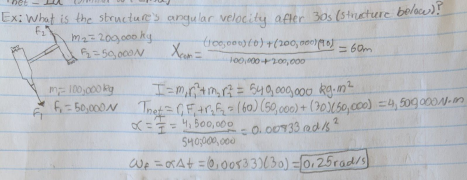12.7 Rotation About a Fixed Axis:

Just putting the equation Tnet =Iα into use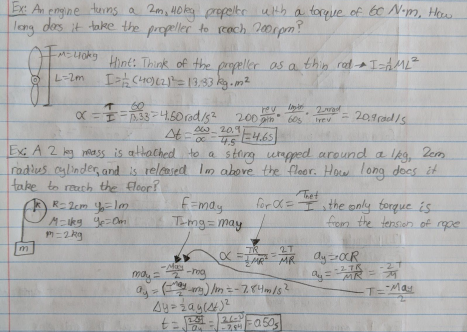12.8 Static Equilibrium:

Just how:

Fnet = 0 >> a = 0

Tnet = 0 >> α = 0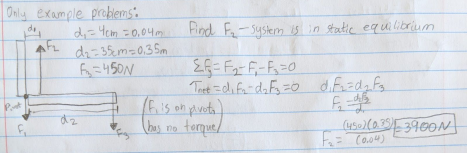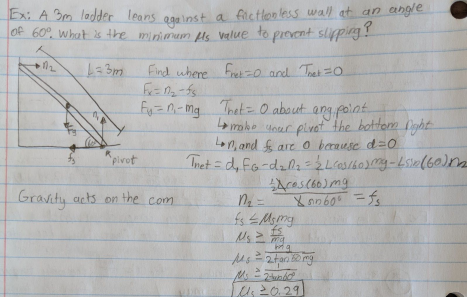12.11 Angular Momentum:

r = particles position

p = mv (momentum as we know from previous chapters)Angular Momentum and Angular Velocity:

L = Iw, in the direction of the right hand rule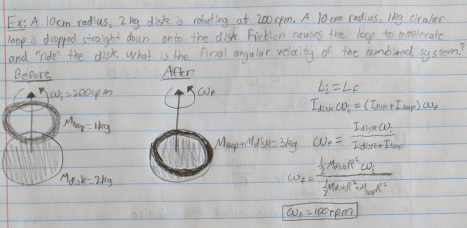____________________________________________________________________________ Chapter 13 - Newton’s Theory of Gravity (really not much to this chapter): 13.3 Newton’s Law of Gravity:

g = v2/r >> v = (gr)^(½)

Two masses create a gravitational force on each other: F1on2 = F2on1 = (Gm1m2)/r2 G = 6.67 x 10-11(Units are Nm2/kg2)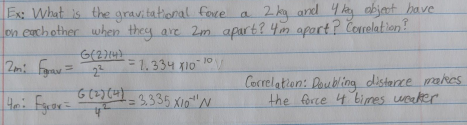13.5 Gravitational Potential Energy:

13.6 Satellite Orbits and Energies (probably won't be asked any of this): F2on1 = (Gm1m2)/r2 = mar = mv2/r

M = more massive body

m = lesser, which is always the satellite

(satellite is just a term for a smaller object that orbits a larger object)

Page ExpiredIt looks like your free minutes have expired! Lucky for you we have all the content you need, just sign up here